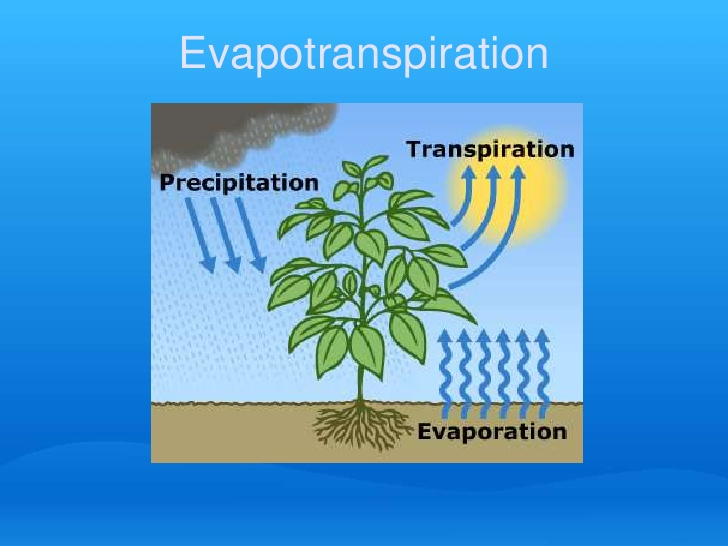Feb 26, 2016

Feb 26, 2016

Feb 26, 2016

Evapotranspiration Measurement by Blaney Criddle & HargreavesBlaney-Criddle Formula

The estimation of potential evapotranspiration is achieved by adopting empirical approaches, such as the Thornthwaite equation, the Blaney-Criddle formula and the Hargreaves method, all having as a requirement the availability of temperature data. The data set is made up of temperature time series, obtained from gauging stations.

The potential evapotranspiration estimated for each station using the above-mentioned methods is spatially integrated, in order to obtain the areal potential evapo-transpiration. The methods adopted for the spatial integration of the point estimates are the Kriging method, the method of Inverse Distance Weighting, the Spline method and the Thiessen method, using applications in a Geographic Information System (GIS) with a spatial resolution of 200x200m2.

u k (k ) (t x p) / 100

TR = kc kt) u = monthly consumptive use (inches)

kt = climatic coefficient

= 0.173 * t - 0.314

kc = crop growth stage coefficient

t = mean monthly air temperature (°F)

p = monthly percentage of annual daylight hours

Hargreaves Method of measurement of Evapotranspiration

Originally developed in 1975

• solar radiation and temperature data inputs
• Updated in 1982 and 1985
• solar radiation estimated from extraterrestrial radiation, RA
• Grass reference ET
• May be used to compute daily estimates, but more accurate over longer time steps: 10-days, monthly

ETo ? 0.0023(Tmax ? Tmin T ?17.8) R

ETo = grass reference ET (mm/day)

Tmax = maximum daily air temperature (°C)

Tmin = minimum daily air temperature (°C)

Tmean = mean daily air temperature = (Tmax + Tmin) / 2

Ra = extraterrestrial radiation (mm/day)

Ra (mm/day) = Ra (MJ/m2/day) / 2.45

• Simple, easy to use
• Data required—maximum and minimum air temperature
• Better predictive accuracy in arid climates than modified Blaney-Criddle
• Max-min temperature difference